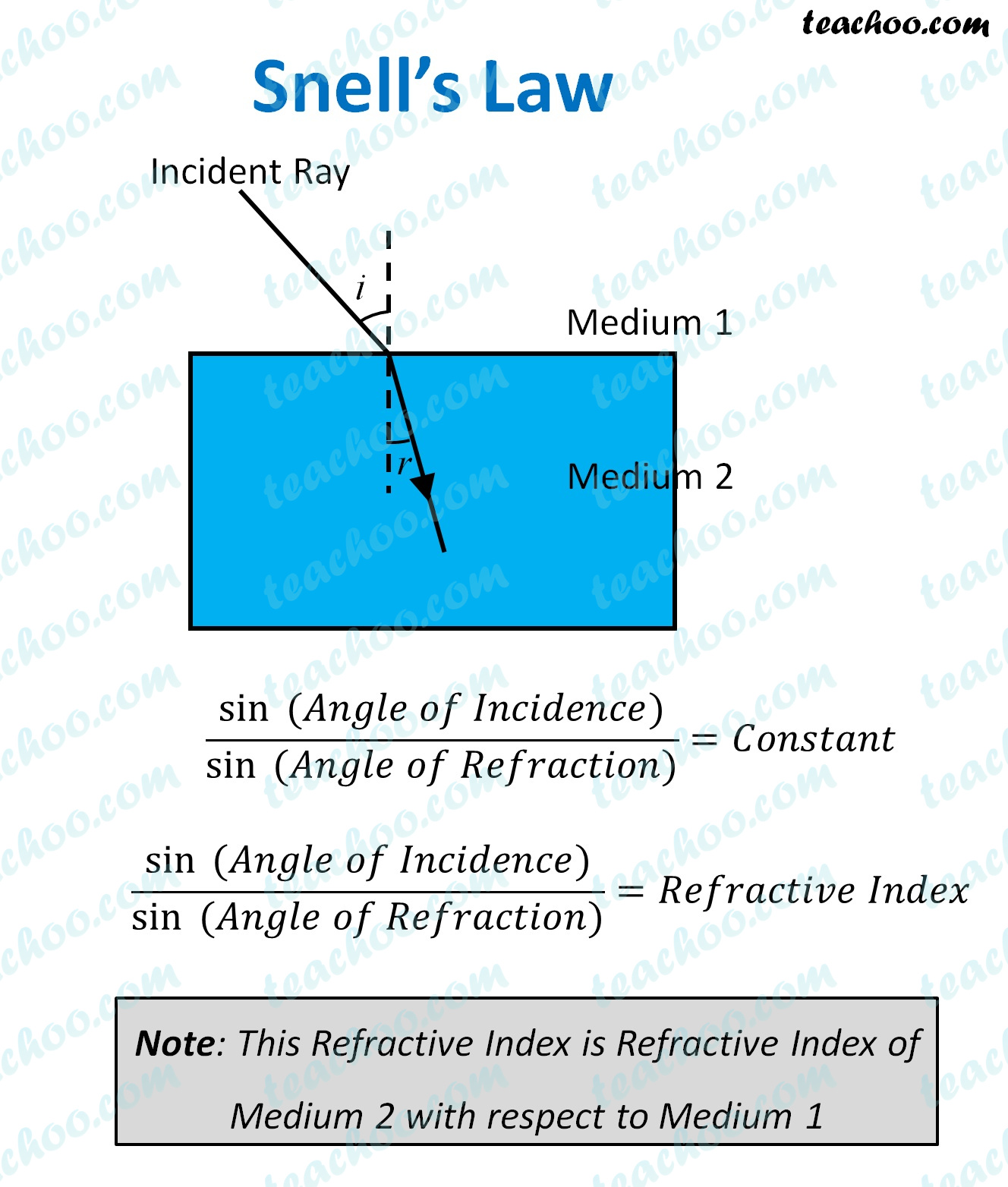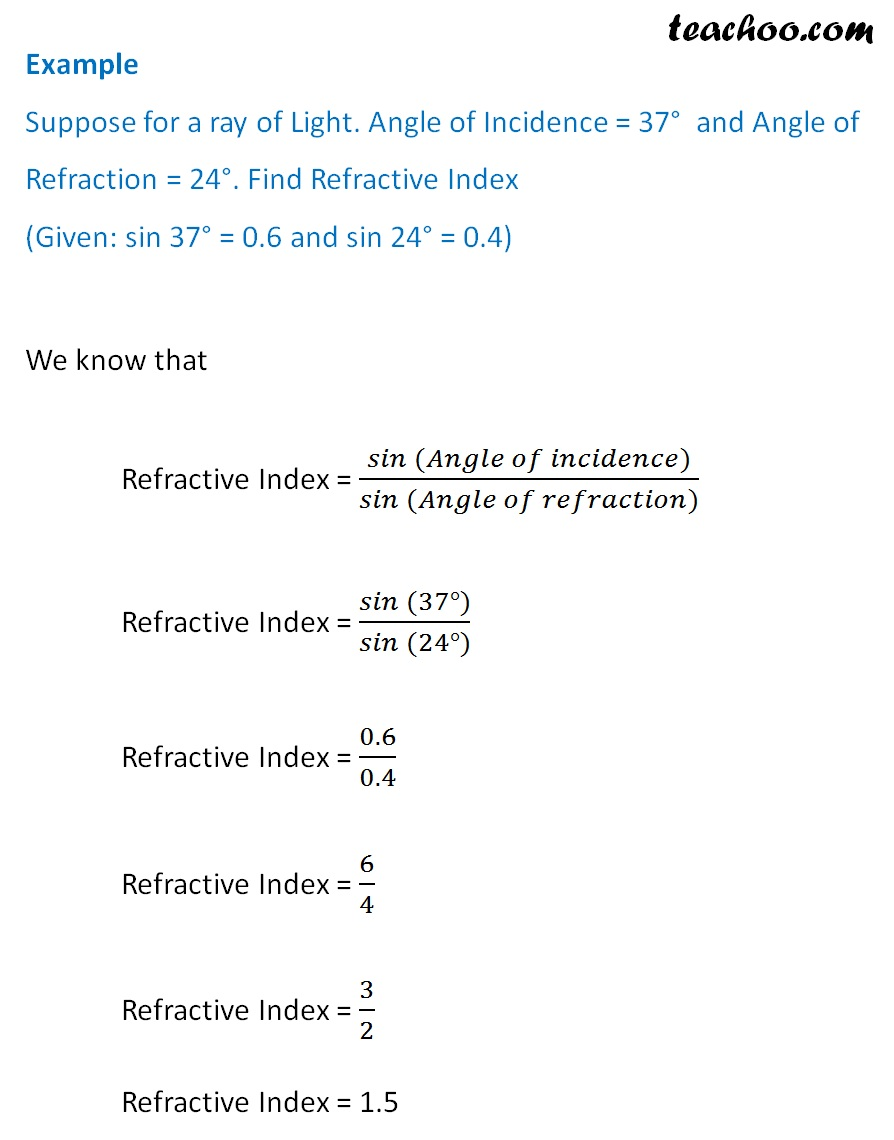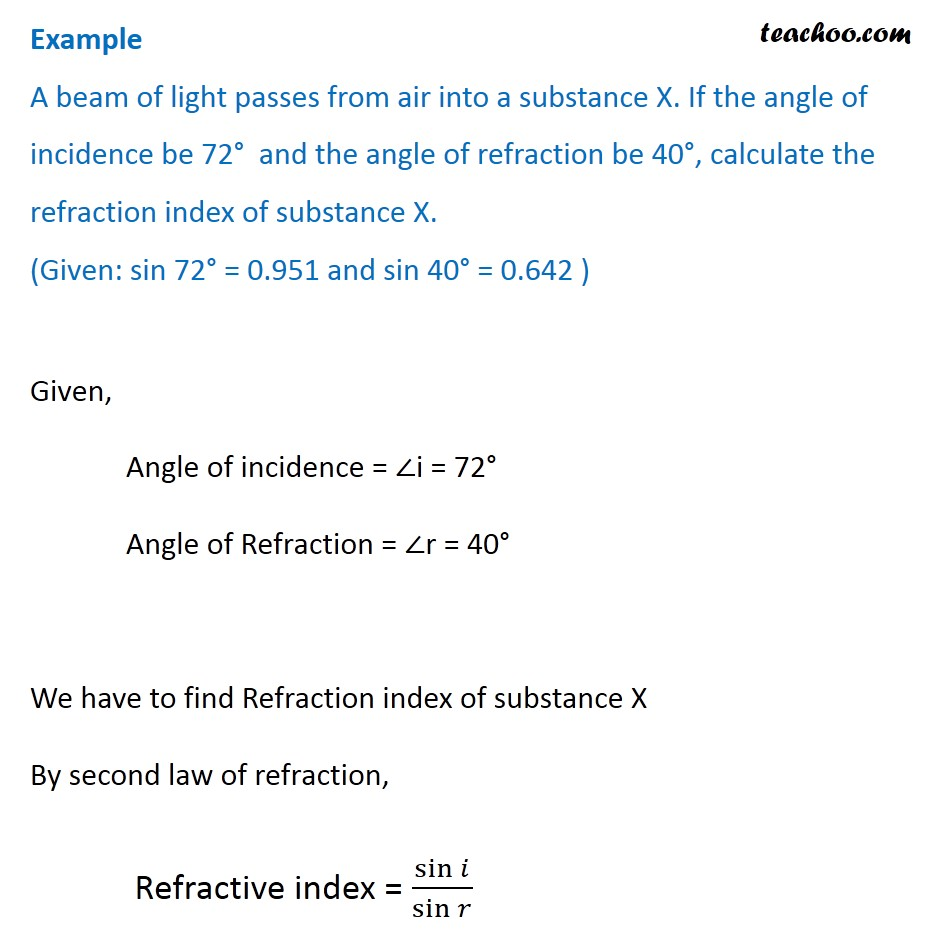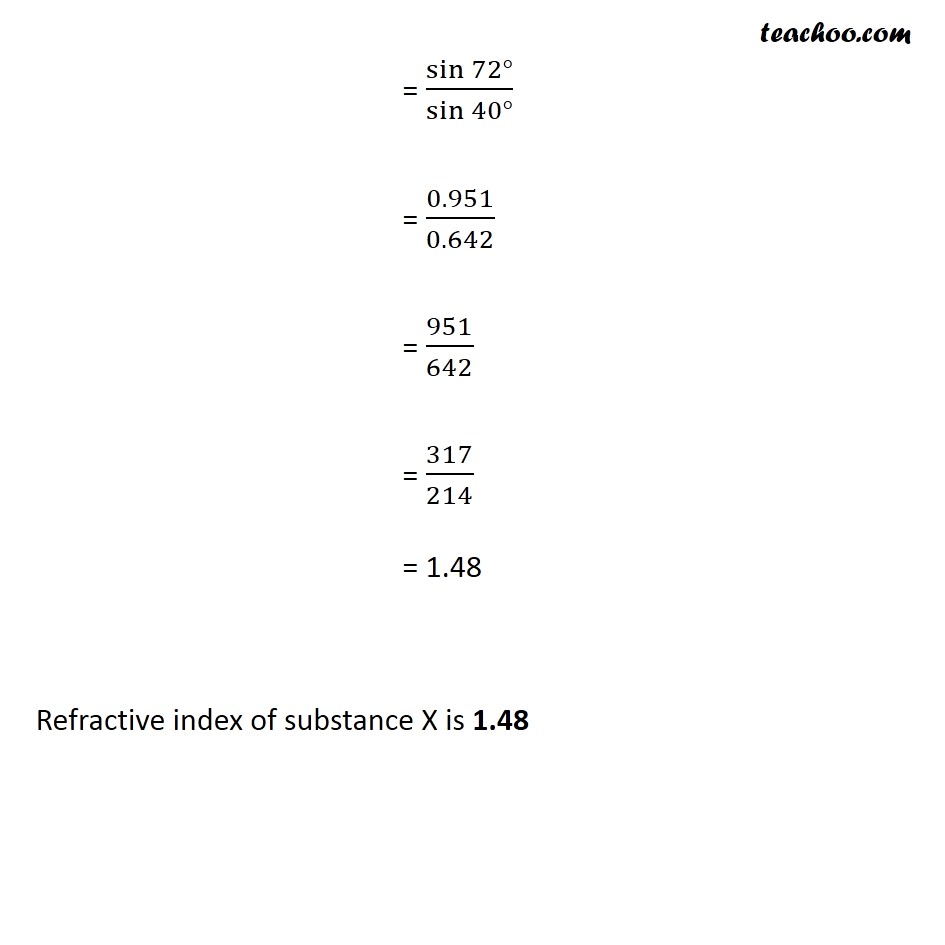Concepts

Class 10
Chapter 10 Class 10 - Light - Reflection and Refraction

There are 2 main laws of Refraction of Light

## Law 1

The Incident Ray,Refracted Ray and Normal Ray all lie on the same plane

## Law 2

The Ratio of sin of Angle of Incidence and Angle of Refraction is constant for a particular medium

This constant is called Refractive Index

It is actually called Refractive Index of the second medium with respect to the first medium.The second law of refraction is also called Snell's Law

Let's do some examples

## Suppose for a ray of Light. Angle of Incidence = 37° and Angle of Refraction = 24°. Find Refractive Index (Given: sin 37° = 0.6 and sin 24° = 0.4)## A beam of light passes from air into a substance X. If the angle of incidence be 72° and the angle of refraction be 40°, calculate the refraction index of substance X. (Given: sin 72° = 0.951 and sin 40° = 0.642 )Learn in your speed, with individual attention - Teachoo Maths 1-on-1 Class

### Transcript

Example Suppose for a ray of Light. Angle of Incidence = 37° and Angle of Refraction = 24°. Find Refractive Index (Given: sin 37° = 0.6 and sin 24° = 0.4) We know that Refractive Index = (𝑠𝑖𝑛 (𝐴𝑛𝑔𝑙𝑒 𝑜𝑓 𝑖𝑛𝑐𝑖𝑑𝑒𝑛𝑐𝑒))/(𝑠𝑖𝑛 (𝐴𝑛𝑔𝑙𝑒 𝑜𝑓 𝑟𝑒𝑓𝑟𝑎𝑐𝑡𝑖𝑜𝑛)) Refractive Index = (𝑠𝑖𝑛 (37°))/(𝑠𝑖𝑛 (24°)) Refractive Index = 0.6/0.4 Refractive Index = 6/4 Refractive Index = 3/2 Refractive Index = 1.5 Example A beam of light passes from air into a substance X. If the angle of incidence be 72° and the angle of refraction be 40°, calculate the refraction index of substance X. (Given: sin 72° = 0.951 and sin 40° = 0.642 ) Given, Angle of incidence = ∠i = 72° Angle of Refraction = ∠r = 40° We have to find Refraction index of substance X By second law of refraction, Refractive index = sin⁡𝑖/sin⁡𝑟 = sin⁡〖72°〗/sin⁡〖40°〗 = 0.951/0.642 = 951/642 = 317/214 = 1.48 Refractive index of substance X is 1.48